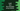# How to find the remainder in swift using modulo# Finding the remainder in swift :

In this tutorial, we will learn how to find the remainder in swift. Swift provides one operator % to find out the remainder. Let’s check how it works :

## Remainder operator :

% is used to find out the remainder. It is also known as modulo operator. This operator is used like below :

``first_number % second_number ``

It will return the remainder after firstnumber_ is divided by the secondnumber_. For example :

``````print(4 % 2)

print(11 % 2)

print(1774 % 3)``````

It will print :

``````0
1
1``````

Note that we can use it only with integers, not with floating-point numbers.